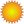cht 電腦資訊 Language C/C++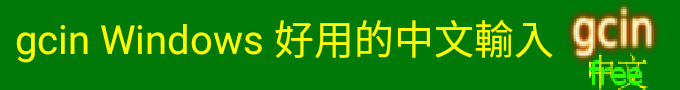# GTK+ 所需要 C 基礎joined: 2007-09-19
posted: 4946
promoted: 325
bookmarked: 206

 1 subject: GTK+ 所需要 C 基礎1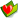1 2008-02-25 quote

# All the C you need to know for GTK+

## A short refresher on basic C concepts

Online on: 2008-02-18

If you want to develop applications with GTK+, a graphical toolkit used by the GNOME desktop environment, it is essential that you are comfortable with the C programming language. This article is meant to give you a short refresher on the basics of C that you will need to know when developing GTK+ applications.

# Basic C program structure

Every C program is composed of one or more functions in the following format. The function receives a number of variable parameters, runs the commands in the function, and then returns a variable of the given type. Note that you can omit the return value by using void as your function type.

```type function (parameters)
{
local variables
commands
}
```

Here is a practical example (don’t worry if you don’t understand it yet):

```int my_function(int a)
{
char b;
b='r';
printf("%d %c",a,c);
}
```

Another example function is shown below. The main() function is the only one that is required by every C program. It receives two parameters (argc and argv), which contain the command line parameters entered when the program begins and the number of parameters. The function should return an integer to exit. The following code will print out “x = 0 and y = 1”. (The printf() function will be covered later in this section!)

```#include <stdio.h>
int main (int argc, char *argv[])
{
int x, y;
x = 0;
printf ("x = %d ", x);
y = 1;
printf ("and y = %d", y);
return 0;
}
```

```gcc -o result result.c
```

You will now be able to run the program by typing ./result in the directory where the program was compiled. It’s handy to have a console open with the program, and a second console with the command line above, ready to compile the code.

## Variable types

C provides a number of variable types. You may notice that these are very basic types, all holding numbers, but they can be used to represent any piece of data. The following table gives an overview of the types that are available to you.

Data Type Bytes Lower Bound Upper Bound
char 1 -128 127
unsigned char 1 0 255
short (int) 2 -32768 32767
unsigned short (int) 2 0 65536
int 4 -2^31 2^31 - 1
unsigned int 4 0 2^32 - 1
long (int) 4 -2^31 2^31 - 1
unsigned long (int) 4 0 2^32 - 1
float 4 -3.2e38 3.2e38
double 8 -1.7e308 1.7e308

C Data Types

It’s important to think about where you place the declaration when creating variables. The scope of a variable refers to which parts of the application can access the variable. In general, variables use the following scope rules in C:

1. You can only access a variable that has been declared before referencing it. In other words, the declaration of a variable must appear above its use.
2. A variable cannot be accessed outside the set of brackets where it was declared. For example, if a variable was declared inside of a for loop, it cannot be accessed outside of that loop.

You can declare variables outside any function, such as above the main() function. This is called a global variable. Also, a variable declared within a function is local to that function.

## Arrays

The variable types previously mentioned are useful, but what if you want a thousand integers? It would take quite some time to create a variable for each of these integers. To solve this problem, you could create an array of integers. The following program creates an array, and then fills it up with the numbers 1 to 1000. Notice that arrays are indexed beginning from zero.

```int arrayOfInt, i;
for (i = 0; i < 1000; i++)
arrayOfInt[i] = i + 1;
```

It is also possible to create arrays with multiple dimensions. The following applications creates an array that has 1000 columns and 1000 rows, and fills it up with the sum of the indexes.

```int multiArray, i, j;
for (i = 0; i < 1000; i++)
for (j = 0; j < 1000; j++)
arrayOfInt[i][j] = i + j;
```

The maximum number of dimensions is defined by the compiler, but most applications do not use more than three at the most. If you reach the maximum (GCC’s maximum is 29), you should consider rethinking your approach to the problem at hand!

## Outputting (printing) data

Although GTK+ is a graphical toolkit, it is still useful to be able to output debugging information to the terminal. To do this in C, you would use printf(), which you already saw in a previous example. You can use additional parameters in this function to embed numbers and strings into the output text. In order for this function to work, you must include the header file stdio.h

The following example would print out “x = 5 and y = 10.7”. The %d is replaced by the first variable, which is an integer. Then, %4.1f is replaced by the second floating point variable with a maximum length of 4 characters and one character following the decimal point.

```int x = 5;
double y = 10.74;
printf ("x = %d and y = %4.1f", x, y);
```

There are a large number of options available of printf(). It would be pointless to enumerate them here since the reference is available on thousands of sites around the Internet. To read more on this function, take a look at this page.

# Conditionals and loops

C provides a number of methods for controlling the flow of your program. In order to understand these, you need to understand a few logical operators that are available. They are introduced in the following table.

Operator Description
== Comparison operator that returns true if the value on the left is equal to the value on the right.
!= Comparison operator that returns true if the value on the left is not equal to the value on the right.
< (>) Comparison operator that return true if the value on the left is less than (greater than) the value on the right.
<= (>=) Comparison operator that return true if the value on the left is less than (greater than) or equal to the value on the right.
&& Used to concatenate multiple comparisons that will only return true if both evaluate to true. For example, ((2 > 1) && (0 > 1)) would return false.
| | Used to concatenate multiple comparisons that will return true if at least one evaluates to true. For example, ((2 > 1) || (0 > 1)) would return true.
! Returns the opposite value of the following expression. For example, !(1 > 0) would return false.

Logical Operators in C

## If/else comparisons

The if statement is a comparison command that can be used to run code only if a condition is met. In addition, you can include optional else if statements, that will only be evaluated if the previous statements were found to be false. Lastly, an else statement can be used at the end to catch all other cases.

The following example shows you how to use an if. Note, the curly brackets can be omitted if only one command is run after a conditional statement.

```int x;
if (x > 0) {
printf ("x is positive");
}
else if (x == 0) {
printf ("x is equal to zero");
}
else {
printf ("x is negative");
}
```

In this example, what would be printed if x was equal to -5, 0, or 5? The application would print “x is negative”, “x is equal to zero”, and “x is positive” respectively.

## The switch statement

The switch can be used as a cleaner style in place of some if statements. It compares the variable or expression in the switch to each case value. If the correct value is found, all of the commands will be run until a break or the end of the statement is reached.

In the following example, white space will be printed if ch is a space, tab, or new line character. If the letter is an uppercase vowel, vowel will be printed. Otherwise, the output will show other character.

```char ch;
switch (ch) {
case ' ':
case '\t':
case '\n':
printf ("white space");
break;
case 'A':
case 'E':
case 'I':
case 'O':
case 'U':
printf ("vowel");
break;
default:
printf ("other character");
}
```

The default case is not required, but it can be used to catch all other cases not previously specified. Note that each case value must be constant, meaning that they cannot be variables.

## While loops

The while loop will continue to run its contained commands over and over until its condition is evaluated as false.

```while (condition) {
commands
}
```

In the following example, the while will continue running until x is greater than or equal to ten. The break statement can be used to exit the loop before it is completed. What will this code print out?

```int x = 0;
while (x < 10) {
printf ("%d ", x);
x += 2;
if ((x % 3) == 0)
break;
}
```

The code above will print 0 2 4 . The x variable is incremented by 2 every time, but when it reaches 6, the if statement evaluates to true and exits the loop. Note: The percent sign (%) represents the modulus operator, which returns the remainder of the division.

## For loops

The for loop allows you to perform initialization, comparison, and incrementing all at once. You can omit one or all of these steps by leaving it blank, although leaving out the comparison will make an infinite loop. In this case, you could use the break command to exit the loop when necessary.

```for (initialize; comparison; increment) {
commands
}
```

In the following example, the integer i is initialized to zero at the beginning of the loop. It will continue running until i is greater than or equal to ten, incrementing by one every time the loop is run. What will this code output?

```int i;
for (i = 0; i < 10; i++) {
if ((x % 3) == 0)
continue;
printf ("%d ", i);
}
```

The above code will output 1 2 4 5 7 8 . If x is divisible by three, continue will skip the rest of the loop’s iteration, so the values 0, 3, 6, and 9 will not be printed. You can also use the break command with for loops.

# Pointers

One of the most important parts the C programming language is the pointer. A pointer is basically a variable that store the memory address of a variable, array, function, etc. There are two operators that are used with pointers:

• The ampersand (&) symbol is called the monadic or unary operator. It returns the address where the variable is located.
• The asterisk (*) symbol is used for dereferencing, and returns the object that is at the location stored by a pointer.

To help you understand this concept, look at the following example. The second line creates a pointer that stores the memory location of x. The next line dereferences ptr, printing out the integer at that memory location (1). Then, the memory location ptr is set to the value 7. Since ptr points to x, the last statement prints out “7”.

If you find this confusing, slowly re-read the sentence above (yes, it does make sense!) and experiment with the code.

```int x = 1;
int *ptr = &x;
printf ("%d", *ptr);
*ptr = 7;
printf ("%d", x);
```

Pointers are very powerful, because they can be used with arrays. The following example creates an array of one hundred characters, and then uses a pointer to traverse the array, setting each to the value of its index.

```char chArray;
char *ptr;
int i;
for (i = 0, ptr = &chArray; i < 100; i++, ++ptr)
*ptr = i;
```

This example shows a few important concepts. First, you should notice that you can provide multiple commands in the first and third parts of the for statement by separating them will commas. This allows you to initialize multiple variables, or provide more than one increment.

In terms of pointers, you can increment or decrement a pointer by using standard integer operations. It is legal to move a pointer with any of the following: ++ptr, --ptr, ptr += 10, ptr -= intVariable, etc.

## Strings

Strings are very important to any programming language, because human interaction would be very difficult otherwise. A string is defined in C as an array of char variables. Each string in C must end with the null character (‘\0’ or 0) so that the end can be found. This is because most strings are stored as pointers.

The following example shows one way to create a string. A pointer called text is created that points to nothing at first. It is then initialized to “Hello world!” and printed to the screen.

```char *text;
text = "Hello world!";
printf (text);
```

This string was initialized by setting the text directly, but this is generally not a good idea. Instead, you should use a function defined in string.h called strdup() to dynamically allocate a copy of the string like the following example. Once the application is done with the string, free() should be called so that the memory taken up by that string can be used by other parts of the program.

```char *text;
text = strdup ("Hello world!");
printf (text);
free (text);
```

There are a number of other functions available in string.h for manipulating strings. The following application shows a few of them, but you should reference the header file for a full list of functions.

```#include <stdio.h>
#include <string.h>
#include <stdlib.h>
int main ()
{
char *text1, *text2;
text1 = strdup ("+");
text2 = (char*) malloc (10 * sizeof (char));
strcpy (text2, text1);
while (strlen (text2) < 10)
{
if (strcmp (text2, "+++++") == 0)
printf ("Pluses: ");
strcat (text2, text1);
}
printf (text2);
free (text1);
free (text2);
return 0;
}
```

The example above is a bit frivolous, but it shows some of the most common functions used for string manipulation. First, the malloc() function was used to allocate a piece of memory with a size of 10 bytes. This function requires you to include stdlib.h and will be covered in more detail in the next section.

Then, the contents of text1 are copied into text2 with strcpy(). The loop continues until the length of text2 is nine characters. During each iteration of the loop, a single plus is concatenated to the variable with strcat().

The conditional statement uses strcmp() to compare two strings. It will return a negative number if the first string should be sorted before the second, zero if the two strings are equal, or a positive number otherwise. You should note that you cannot use ==, !=, or any other operator to compare strings, since using those operators would just comparing the pointer location!

## Dynamic memory allocation

One of the main reasons for pointers is to enable dynamic memory allocation, or the ability to allocate memory while the application is running. Memory is allocated in C with malloc(), which uses the following syntax. It accepts the number of bytes to allocate, returning a pointer to the newly allocated memory location. When you are done with the memory, you must call free(), or that space will be leaked by your application!

```int *data;
data = (int*) malloc (100 * sizeof (int));
```

In addition to this function, you can use calloc(), which allocates an array of data with the given size. In the following example, an array of 100 elements with a size of 4 bytes is allocated. This code does the same essentially the same thing as the previous example.

```int *data;
data = (int*) calloc (100, sizeof (int));
```

As with malloc(), everything allocated with calloc() should be freed when you are done using it. Also, one of the most common problems encountered with pointers is not allocating memory before using it, so make sure to avoid using uninitialized pointers! Basically, if you get a Segmentation Fault, you are misusing pointers.

# Structures

The struct type allows you to create your own data types in C. For example, let us assume you want to store information about an animal. You could use the following structure to do this:

```typedef struct
{
char *name;
char *sound;
int legs;
} animal;
```

The structure definition above creates a new data type called animal. This data type holds two strings and one integer. This is useful because you can create as many instances of animal as you want. The following code shows two ways you can use the new structure, one without and one with pointers.

```animal cow;
animal *chicken;
cow.name = strdup ("Cow");
cow.sound = strdup ("Moo");
cow.legs = 4;
chicken = (animal*) malloc (sizeof (animal));
chicken->name = strdup ("Chicken");
chicken->sound = strdup ("Cluck");
chicken->legs = 2;
free (chicken);
```

In this example, and instance of animal is created called cow. Since this is not a pointer, you can use the period character to reference the members of the structure. In the second part of this code, the memory is allocated for another animal instance. Notice that, with a pointer, you must use -> to access the members of the structure!

It may not be immediately apparent from this example why you would want to use a pointer. However, if you wanted to pass the structure to a function, it would be much more convenient. Passing chicken would just create a copy of the pointer. This is why pointers are preferred in C.

# Conclusion

By now, you should be familiar with the basics of the C programming language. While there are other aspects of the language that you may need to learn when programming with GTK+, these basics will allow you to use all but the most advanced portions of the libraries.

If you are interested in learning GTK+, I would encourage you to check out my book on the subject: Foundations of GTK+ Development. This book covers everything from the basics of the libraries to creating your own widgets, and much more in between.

(C) Andrew Krause 2007

Verbatim copying and distribution of this entire article is permitted in any medium without royalty provided this notice is preserved.

# Biography

Andrew Krause: Andrew Krause is the author of Foundations of GTK+ Development (www.gtkbook.com). He is an active contributor to the Open Source community and is currently majoring in Computer Engineering at Penn State University. Andrew will be working for Argon ST as a software engineer beginning in May of 2008.

edited: 1
qrtt1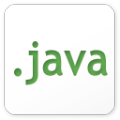joined: 2007-10-02
posted: 82
promoted: 18
bookmarked: 5

 2 subject:00 2008-02-27 quote

http://gcc.gnu.org/onlinedocs/cpp/joined: 2007-09-19
posted: 4946
promoted: 325
bookmarked: 206

 3 subject:00 2008-02-27 quote

edited: 1
qrtt1joined: 2007-10-02
posted: 82
promoted: 18
bookmarked: 5

 4 subject:00 2008-03-01 quote

(逃~~~~~~~~~)

carl_tw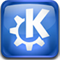joined: 2008-05-04
posted: 71
promoted: 0
bookmarked: 0
Taipei, Taiwan
 5 subject:00 2008-05-05 quote

guest
 6 subject:00 2008-05-06 quotecht 電腦資訊 Language C/C++